Browse by Topic

# Chemistry Step-by-Step Solutions: Chemical Reactions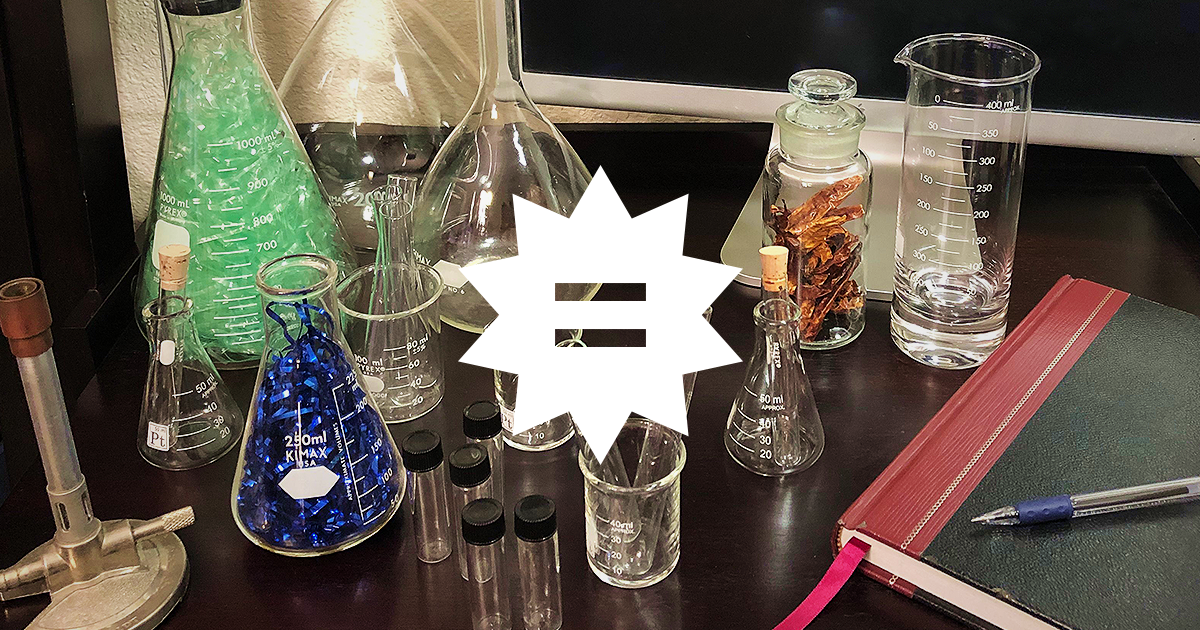If you’re studying chemistry or are in a discipline requiring chemistry prerequisite courses, then you know how expensive the required textbooks can be. To combat this, the chemical education community has developed open educational resources to provide free chemistry textbooks. However, although free textbooks keep cash in your wallet, they don’t include solution guides for all the homework problems.

Luckily, the Step-by-Step Solutions feature of Wolfram|Alpha has got your back! Whether you’re studying remotely or collaborating via video conferencing, Wolfram|Alpha helps you learn and apply the problem-solving frameworks for chemical word problems. The step-by-step solutions provide stepwise solution guides that can be viewed one step at a time or all at once. The guides not only hone efficient problem solving, but also facilitate digging deeper into concepts that might still be murky.

Over the next few weeks, we’ll be exploring some of the popular topics that middle-school, high-school and college students encounter in their chemistry courses and final exams: chemical reactions, structure and bonding, chemical solutions, and finally, quantum chemistry. Read on for example problems in chemical reactions and their step-by-step solutions!

## Balancing Chemical Equations

A fundamental aspect of chemistry is balancing chemical equations. If chemical equations are the language in which chemical processes are expressed, then balancing chemical equations is the corresponding grammar. The step-by-step solution walks you through a robust algebraic approach to identifying the stoichiometric coefficients.

### Example Problem

Write the balanced equation for the reaction of copper with nitric acid to produce copper nitrate, nitrogen oxide and water.

### Step-by-Step Solution

For this class of problem, just enter “balance copper + nitric acid -> copper nitrate + nitrogen dioxide + water”.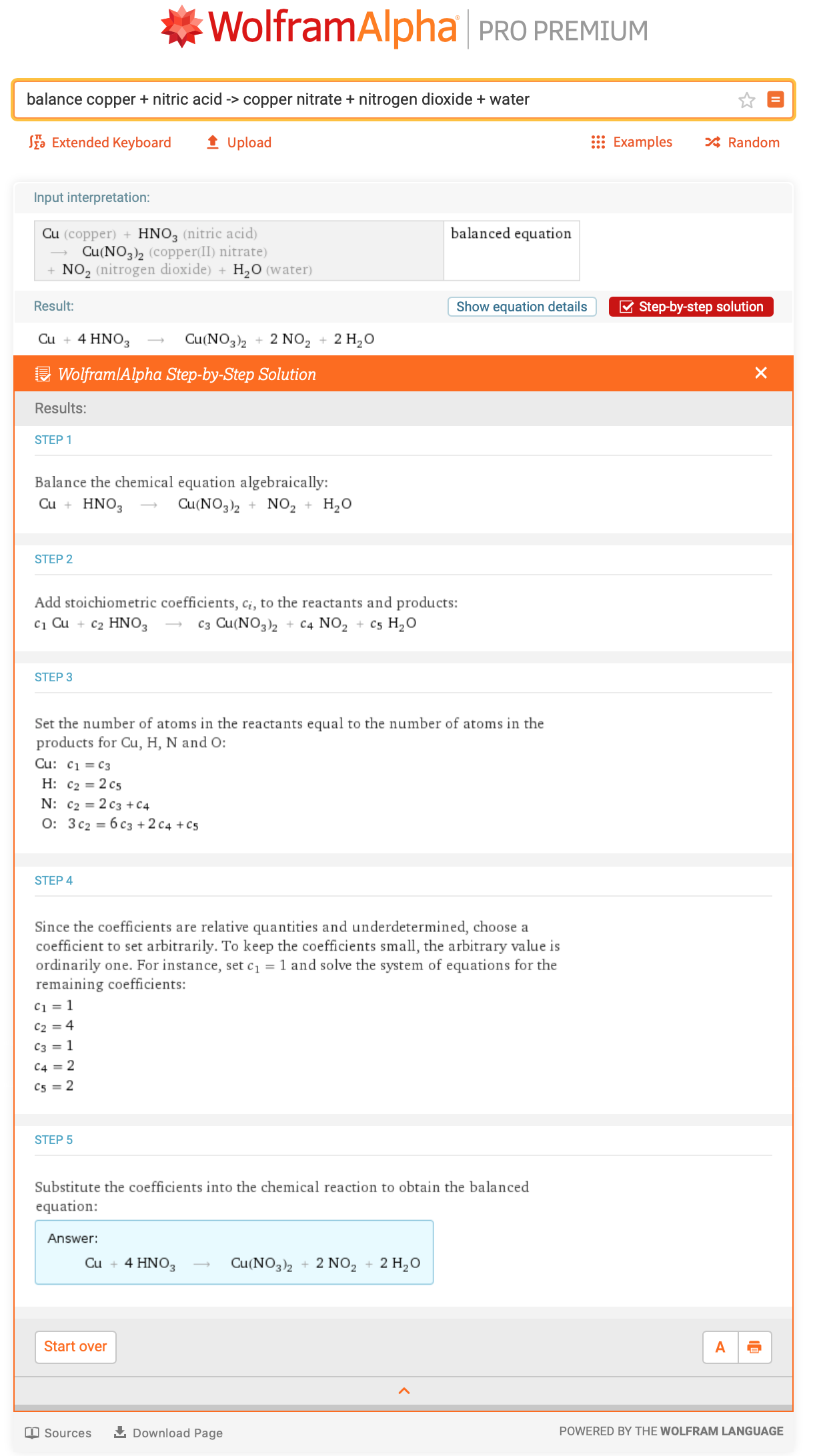## Mass

After balancing the related chemical equations, the next step in planning a laboratory experiment is computing how much of each reactant must be measured out. To do this, one needs the molar mass for each reactant. Step-by-step solutions are available for the molecular mass and relative molecular mass in addition to the molar mass. In all cases, a general framework for solving these types of problems is provided via the Plan step. Details of which formula to use and how to gather the necessary information are provided.

### Example Problem

Calculate the molar mass of silver sulfate, Ag2SO4.

### Step-by-Step Solution

In this case, just enter “molar mass silver sulfate”.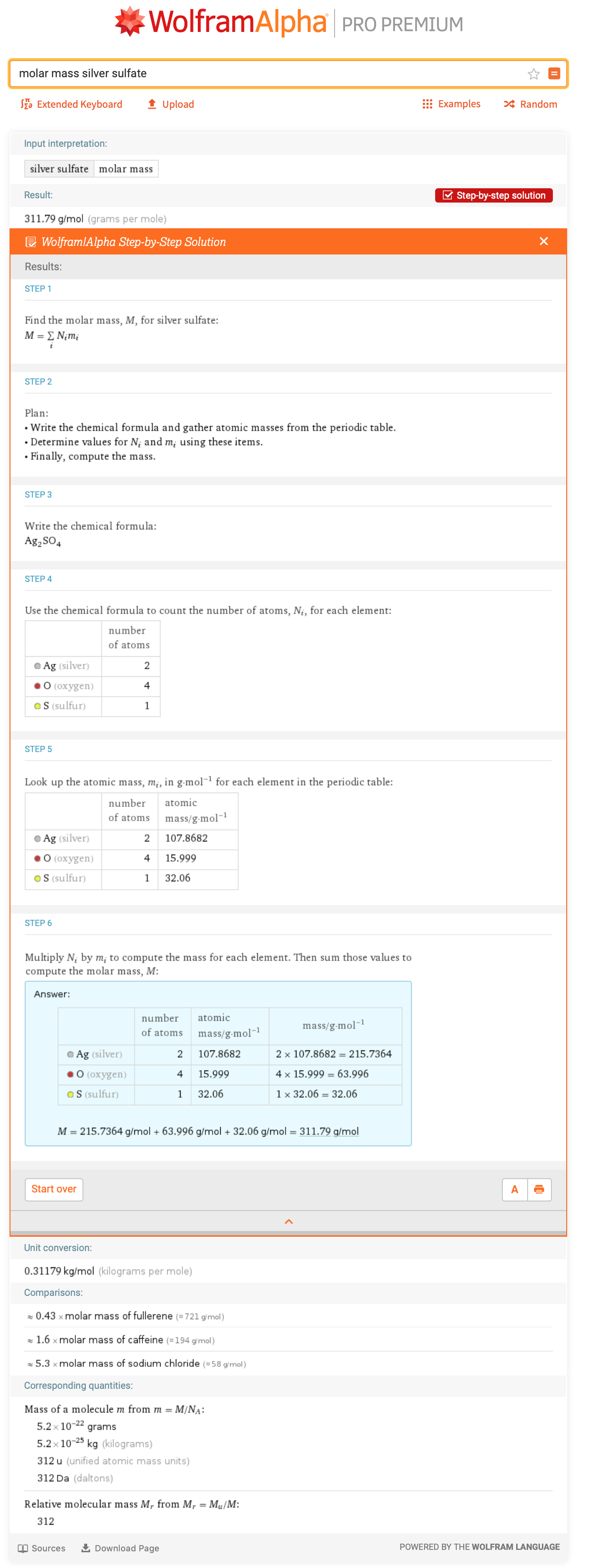## Mass Composition

One way to analyze individual chemicals is to compute and compare the mass and atom percentages. The step-by-step solution provides a general framework for solving this class of problem in the Plan step. Details of the relevant equations, as well as how to compute the necessary intermediate values, are provided. Ways in which you can check your work during the calculations are also available via the “Show intermediate steps” buttons.

### Example Problem

Antihemophilic factor is a coagulant with the formula C11794H18314N3220O355S83. What is its percent composition?

### Step-by-Step Solution

For the answer, just enter “antihemophilic factor elemental composition”.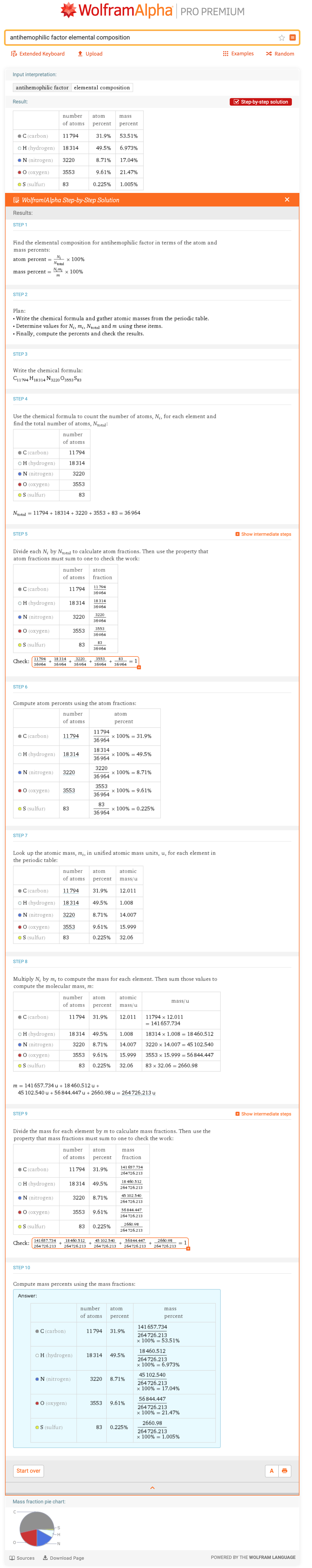## Chemical Conversions

Chemical conversions crop up in nearly every chemistry homework or research problem. As such, step-by-step solutions are available for converting among moles, mass, volume, molecules and atoms. Unit conversions and dimensional analysis details are provided.

### Example Problem

How many atoms are in five milliliters of a 1.5 mM magnesium hydroxide solution?

### Step-by-Step Solution

To solve this, just enter “convert 5 mL of 1.5 mM magnesium hydroxide to atoms”.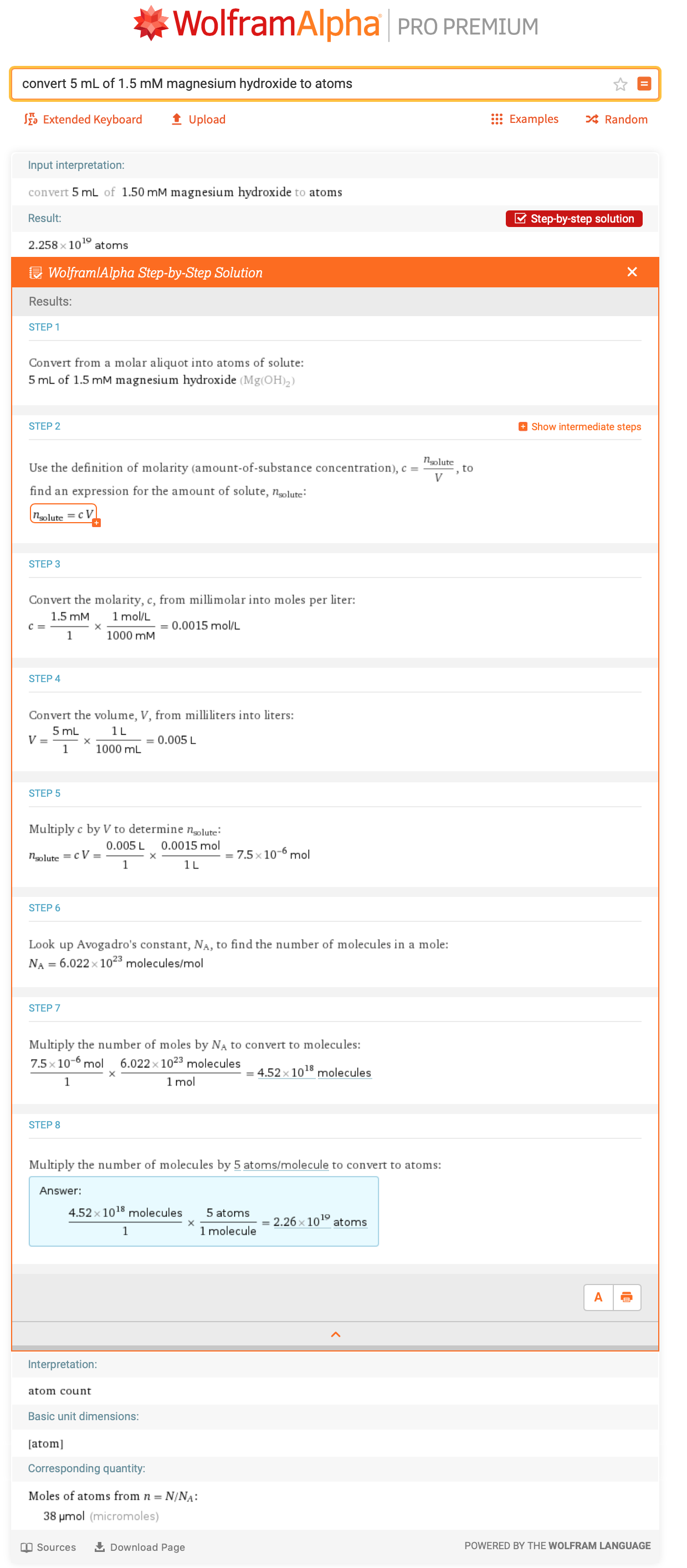## Stoichiometry

After running a chemical reaction, one often wants to know how the reaction went by computing the reaction yields. Step-by-step solutions are available for computing the amount of reactants needed and the theoretical yield in addition to the percent yield. The use of stoichiometric factors to generate the desired values is explained in detail.

### Example Problem

Upon reaction of 1.274 grams of copper sulfate with excess zinc metal, 0.392 grams of copper metal was obtained according to the following equation: CuSO4(aq)+Zn(s)⟶Cu(s)+ZnSO4(aq). What is the percent yield?

### Step-by-Step Solution

To find the percent yield, just append the mass values to the corresponding chemical species and ask for the stoichiometry, “1.274 g CuSO4 + Zn -> 0.392 g Cu + ZnSO4 stoichiometry”.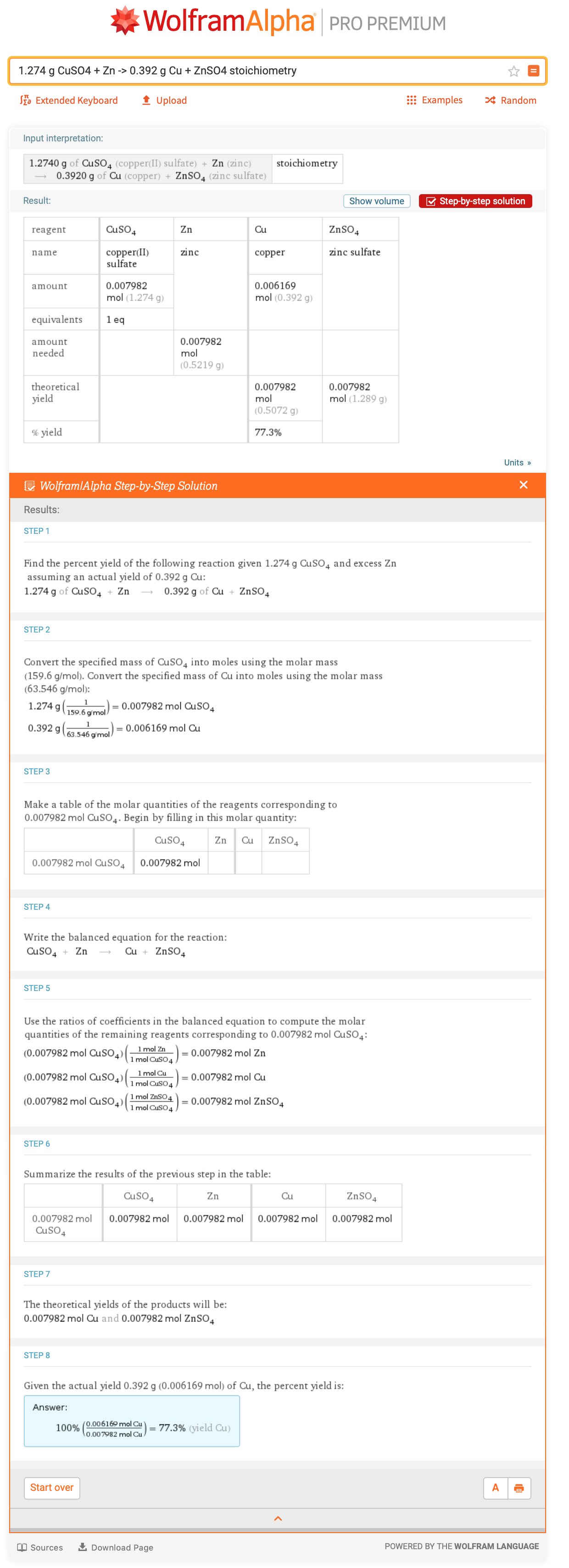## Challenge Problems

Test your chemical reaction problem-solving skills by using the Wolfram|Alpha tools described to solve these word problems. Answers will be provided in the next blog post in this series.

1. Compute the molecular mass of acetaminophen. Is the element with the largest atom count also the element with the largest mass percent?
2. What is the limiting reactant and theoretical yield when 24.8 grams of white phosphorus and 0.200 moles of oxygen react to form 10.0 grams of phosphorus pentoxide?

## And More Chemistry to Come

Whether you’re studying for upcoming final exams, puzzling out homework or just looking for a refresher, chemical reactions are one of many chemistry topics covered by the Wolfram|Alpha knowledgebase. Next week we’ll cover step-by-step solutions for chemical solutions, followed by structure and bonding, and then quantum chemistry. If you have suggestions for other step-by-step content (in chemistry or other subjects), please let us know! You can reach us by leaving a comment below or sending in feedback at the bottom of any Wolfram|Alpha query page.